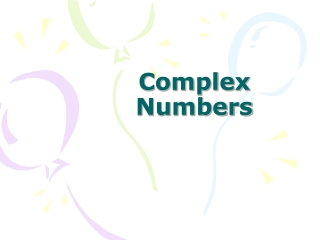DownloadDownload PresentationComplex Numbers

# Complex Numbers

Download Presentation## Complex Numbers

- - - - - - - - - - - - - - - - - - - - - - - - - - - E N D - - - - - - - - - - - - - - - - - - - - - - - - - - -
##### Presentation Transcript

1. Complex Numbers

2. Imaginary Unit • Until now, you have always been told that you can’t take the square root of a negative number. If you use imaginary units, you can! • The imaginary unit is ¡. • ¡= • It is used to write the square root of a negative number.

3. Property of the square root of negative numbers • If r is a positive real number, then Examples:

4. *For larger exponents, divide the exponent by 4, then use the remainder as your exponent instead. Example:

5. Examples

6. Complex Numbers • A complex number has a real part & an imaginary part. • Standard form is: Real part Imaginary part Example: 5+4i

7. The Complex plane Real Axis Imaginary Axis

8. Graphing in the complex plane

9. Adding and Subtracting(add or subtract the real parts, then add or subtract the imaginary parts) Ex: Ex: Ex:

10. MultiplyingTreat the i’s like variables, then change any that are not to the first power Ex: Ex:

11. Absolute Value of a Complex Number • The distance the complex number is from the origin on the complex plane. • If you have a complex number the absolute value can be found using:

12. Examples 1. 2. Which of these 2 complex numbers is closest to the origin? -2+5i